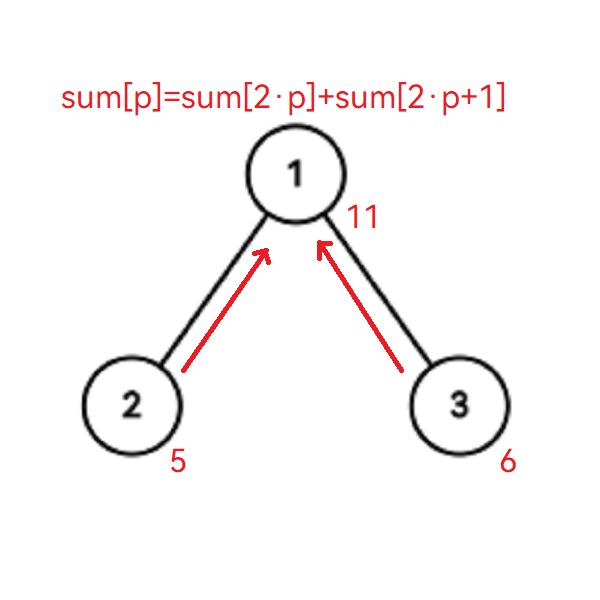## 线段树基本结构• $1.$ 线段树的每一个节点都管理着一段区间，其值为这一区间内需要维护的值，根节点（编号为 $1$ ）管理着整段区间 $[1,n]$ ，叶子节点管理的区间长度为 $1$ 。

• $2.$ 除叶子节点外，其他节点分别有两个子节点，如当前节点的编号为 $p$ ,则左子节点的编号为 $2p$ ，右子节点的编号为 $2p+1$ 。

• $3.$ 如节点 $p$ 的管理区间为 $[l,r]$ ，设此区间的中点为 $mid$ ，则左子节点管理区间的范围是 $[l,mid]$ ，右子节点管理区间的范围是 $[mid+1,r]$ 。

## 线段树建树## 线段树单点修改

• $1.$ 从根节点出发，往下寻找目标节点，修改其值。

• $2.$ 将总和回传到根节点。## 线段树区间查询

• $1.$ 当前节点的区间包含在查询区间之内，直接返回当前节点的 $sum$ 值。
• $2.$ 当前区间与左子树区间有重叠部分，那么递归搜索左子树，加上答案。
• $3.$ 当前区间右子树区间有重叠部分，那么递归搜索右子树，加上答案。## 线段树区间修改“懒”标记指的是当前节点修改过了，但没有让下边的节点修改的一种标记，可以再查询时需要时下传，大大节省时间，所以是懒标记。